## Step to Build Email Spam Classification Model

By: Ram on Jul 24, 2022

We get hundreds of emails every day and not all the emails are relevant to us. We often get irritated with the irrelevant emails and wish to segregate the spam emails from the genuine ones. Machine Learning algorithms can do this job and quite effectively.

In this blog, we will consider a sample dataset and develop Spam Classification Model.

An email contains a few information and ideally, we can consider all of them to classify an email to Spam or not. Key email attributes are:

• Sender Name & Email ID
• Subject
• Content
• Attachment (optional) - Attachment may have file name, extension etc that may be used.

This is an example; the data is textual, and we need to leverage Natural Language Processing (NLP) for developing Machine Learning Model. We will discuss some of the concepts in the relevant sections.

Overall approach to develop the model are:

1. Python Packages
2. Data & Reading Data
3. Exploratory Data Analysis & Feature Engineering
• Summary Statistics & Information
• Label Variable & Distribution
• Text Processing & Word Cloud
4. Model Development
• Text Data manifestation using Tf-IDF
• Split Data
• Model Development - Naive Base
• Model Validation
5. Concluding thoughts and next steps

## 1. Python Packages

`import os`

`import email`

`import random`

`import email.policy`

`from bs4 import BeautifulSoup`

`import numpy as np`

`import pandas as pd`

`from wordcloud import WordCloud, STOPWORDS`

`import matplotlib.pyplot as plt`

`import re`

`import string`

`import tensorflow as tf`

`from tensorflow.keras.preprocessing.text import Tokenizer`

`from sklearn.feature_extraction.text import CountVectorizer`

`from sklearn.feature_extraction.text import TfidfVectorizer`

`from tensorflow.keras.preprocessing.sequence import pad_sequences`

`from sklearn.metrics import classification_report, confusion_matrix, accuracy_score`

`from sklearn.model_selection import train_test_split`

`import sklearn`

`from nltk.corpus import stopwords`

`import nltk`

`from textblob import TextBlob`

`nltk.download('stopwords')`

`stop_words = stopwords.words('english')`

`from nltk.stem import WordNetLemmatizer`

`wordnet_lemmatizer = WordNetLemmatizer()`

`nltk.download('wordnet')`

`nltk.download('punkt')`

`#Import Gaussian Naive Bayes model`

`from sklearn.naive_bayes import GaussianNB`

## 2.Data & Reading Data

We need to develop a ML model to classify emails into Spam and Non-Spam. For our algorithm to know which emails look like Spam and how these are different from the non-Spam email, we need emails data along with their labels (whether Spam or Non-Spam)

There are number of Spam Label dataset available for us to use. In this example, we are using a dataset available on Kaggle.

Dataset Source

We can download and read the data. There are two folder spam and ham in the downloaded zipped folder. Also, we need to read each of the email saved in the respective folder.

We have used some of the python functions from this workbook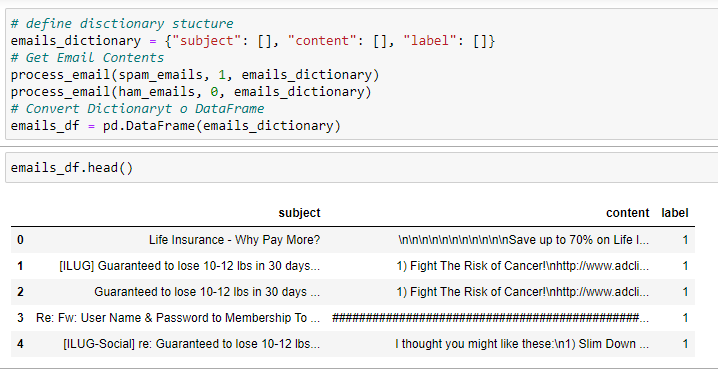## 2.Exploratory Data Analysis & Feature Engineering

Now, we have the dataset with us, and it is always important to carry out Exploratory Data Analysis (EDA). There may be some insights and idea we get to improve the data.

For a classification problem like Spam Classification, we must always check the label data distribution. We can visualize spam/ham emails using a bar chart.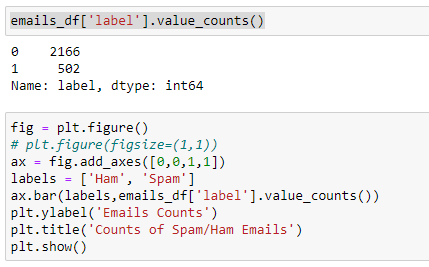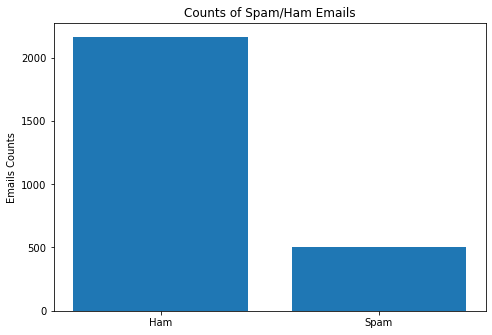### Text Data Pre-processing: Emails

Since, we have text contents for each of these emails. We need to clean up the text data and some of the basic steps are

• Convert to lowercase
• Remove stop words
• Remove non relevant contents such as https
• Convert words to their base form – lemmatization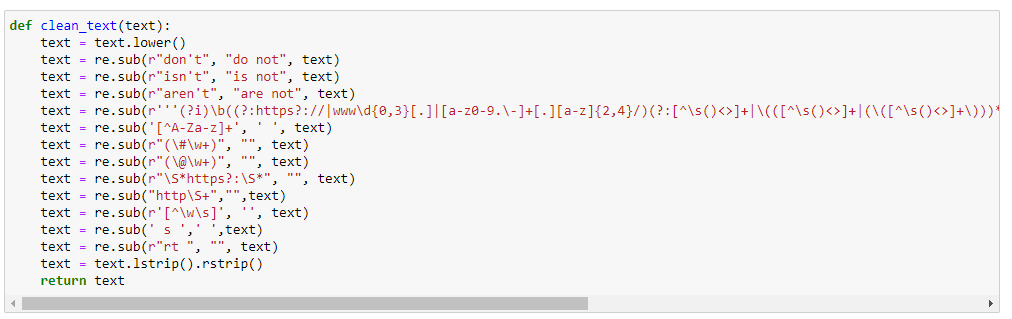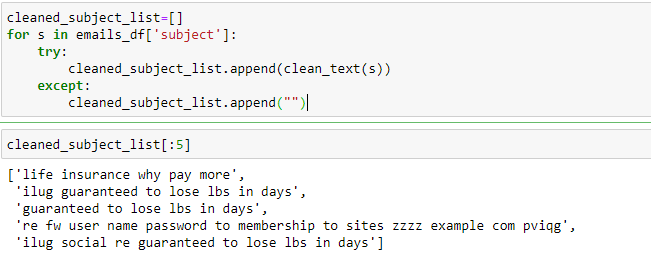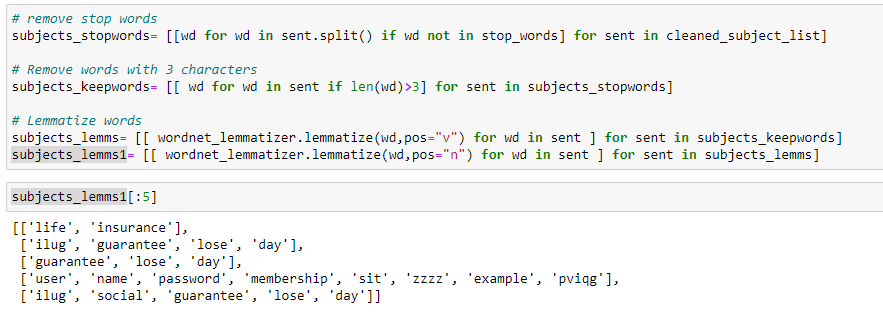Once, we have cleaned text contents and the data is list of words for each of email subject and then list of subjects for the emails.

We have below steps, before create word clouds

• find bi-grams – combinations of words occurring across emails
• Convert to word combinations
• Find counts for each of the combinations (bi-grams) across emails
• Create dictionary – bi-gram and frequency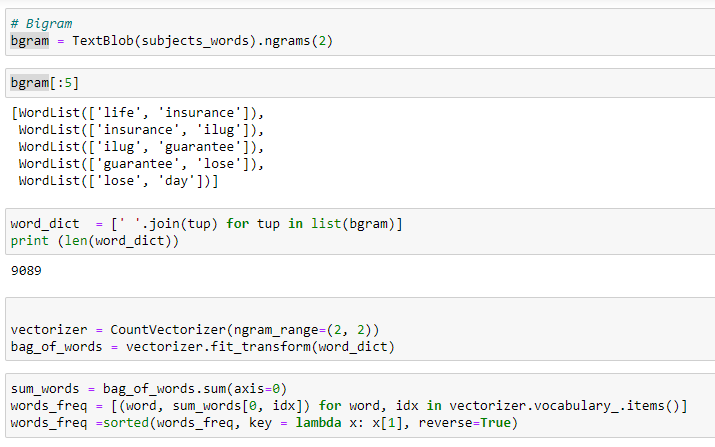### Word Cloud

Word Cloud helps in understanding the common words across the text corpus. For developing the word cloud, we need to find words and their frequencies. Then plot the relative importance using word cloud. So, we need to find NGram of the text documents.

fig = plt.figure(figsize=(10,10))

words_dict = dict(words_freq[:200])

wordCloud = WordCloud(max_words=100, height=350, width=350,stopwords=stop_words)

wordCloud.generate_from_frequencies(words_dict)

plt.title('Word Cloud - Email Subjects')

plt.imshow(wordCloud, interpolation='bilinear')

plt.axis("off")

plt.show()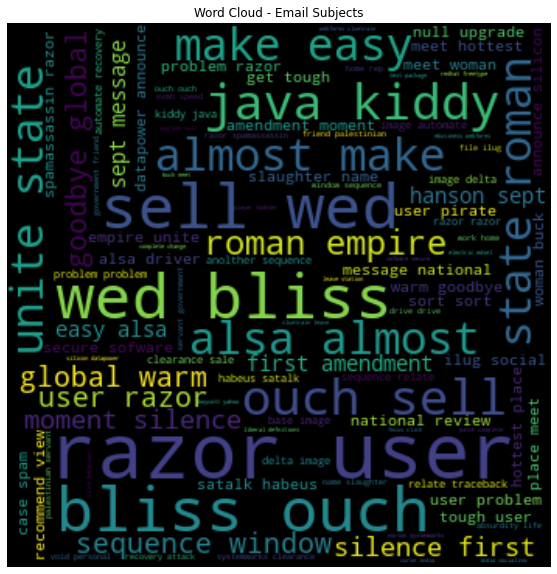We can create separate word clouds for Spam and Ham Emails

## 4. Model Development

### TF IDF

For the model, the input data should be representation numerically. In this case we will convert the subjects’ textual information to a numerically format. For now, the data will be converted into either Tf-IDF vectors or word vector count. We will use Tf-IDF Vectorizer on the email subject data.

TF-IDF Vectorizer creates Tf-IDF values for every word in the email subjects. Tf-IDF values gives a higher value to words appearing less frequently so that commonly occurring words don’t give importance to them but gives more importance to the meaningful and interesting terms used in the email subjects

Term frequency (TF) = (Number of Occurrences of a word)/(Total words in the document)

IDF(word) = Log((Total number of documents)/(Number of documents containing the word))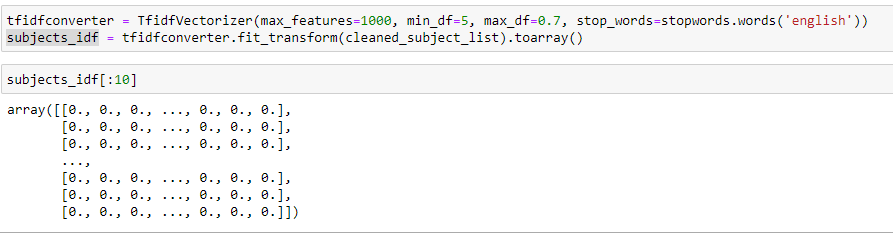### Model Samples/Split Datta

`# labels`

`y = emails_df['label']`

`X_train, X_test, y_train, y_test = train_test_split(X_idf, y, test_size=0.2, random_state=0)`

`print("Length of Train", len(X_train))`

`print("Length of Train", len(X_test))`

`Length of Train 2134`

`Length of Train 534`

Training Text Classification Model - Spam Vs Ham

We can use any of the supervised ML method that can be used for classification. Let's first fit our Naïve Bayes model, on the Tf-IDF vector-based x_train and labelled sample - y_train.

`#Create a Gaussian Classifier`

`modelNB = GaussianNB()`

`# Train the model using the training sets`

`modelNB.fit(X_train,y_train)`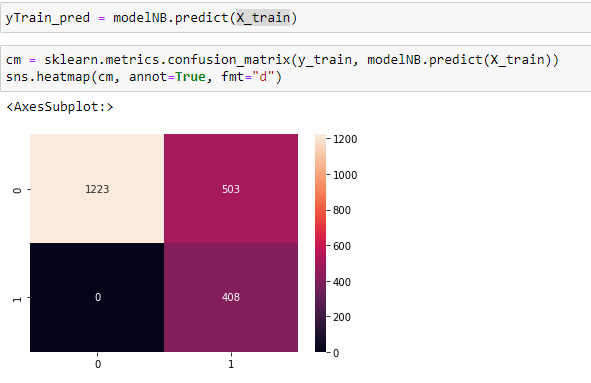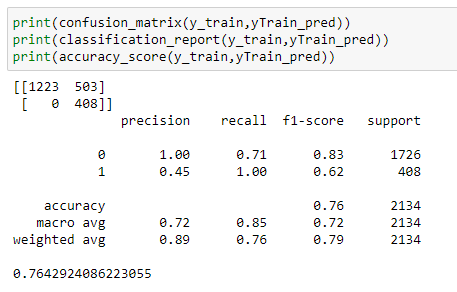Precision tells us % of cases correctly identified. For the emails predicted as Spam, % of these are Spam. In the above confusion matrix, 45% of the emailed identified by the model as Spam are correctly classified.

Recall is termed as capture rate. In this example, % of all the Spam emailed captured by the model. In the above example, all the Spam emailed are identified. So, for Spam (label =1), the call is 1.

Since Precision is only 45% for the Spam class, we can find ways to improve the model.

More information on Precision & Recall - Blog

## Model Enhancements

• Balance Data
• Try other Techniques - LSTM, XGBoost
• Augment Data - Use subject and content

### Balance Data

The current data sample is im-balanced -both classes for the label variable is not equal. in this sample, Spam emails are 551 but Ham emails are 2551. We can make the data balanced and then train the model.

### ML Techniques

We can explore different ML techniques such as SVM, XGBoost or LSTM.

### Augment Data

Currently, we have used only subjects of emails. We can also use the content of the emails. Also, we can create additional features from the subject and content.

## Concluding thoughts and next steps

We have learnt steps to develop an email classification model that can classify emails into Spam or Ham. You can follow the steps to use email content and subject (both together) to improve the accuracy of the model.

Also try any or all the above suggested steps to improve the model.

Full Code

Tags
Most PopularJun 18, 2020Jul 23, 2020Jun 19, 2020Jun 19, 2021